# Quantum cryptography

By Andreas J. C. Woitzik & Stefan Seegerer. Posted

Figure 4 A self-made physical representation of a qubit Credit: Andreas J. C. Woitzik

Originally published in Hello World Issue 18: Cybersecurity, March 2022. All information true at the time of original publishing.

### Andreas J. C. Woitzik and Stefan Seegerer share an activity for older pupils exploring the fundamentals of quantum cryptography and how to detect eavesdropping

We rely on secure communication for a lot of tasks, from private chat to Covid certificates. Fortunately, we have asymmetric encryption protocols such as RSA, where people do not need to have some kind of secure channel to exchange an asymmetric key. However, the rise of quantum computers means that a lot of cryptography systems are now more prone to deciphering, as these computers can decode some security protocols easily. New protocols therefore need to be invented, and interestingly, quantum information can also be used for encryption itself. These quantum protocols enable secure communication by noting a possible interference by an eavesdropper.

One such protocol is BB84, which is fun to discover with students. Before introducing it, students should already have knowledge of one-time pad and classical ciphers such as Caesar, as the secure cryptography protocol motivates the quantum key exchange. Asymmetric cryptography and potential threats to breaking encryption through Shor’s algorithm are also useful prerequisites to introducing this protocol.

### The fundamentals

The BB84 protocol is built upon two key features of quantum information, which are strikingly different to classical information:

• Invasive measurements: Quantum information, in general, is modified when measured.

• No-cloning theorem: Quantum information, in general, cannot be copied.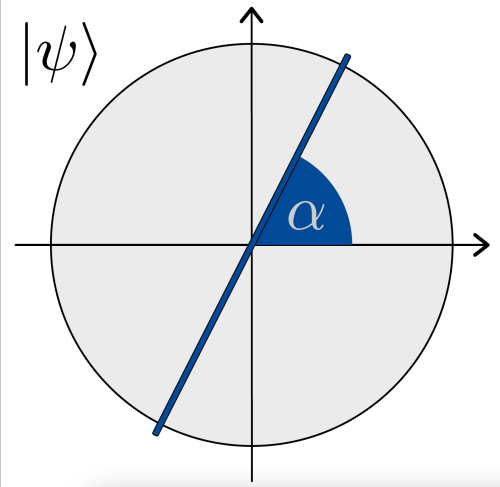Before we look at these features, we need to understand how we can describe quantum information. The fundamental bit of quantum information is a qubit, which is visualised here as a straight line crossing the origin on a unit circle (see Figure 1). The state of a qubit can be any such line that crosses the origin at any angle (meaning that there are infinitely many different states for a single qubit; you can simply rotate it). Usually, we define the x-axis as a 0° angle (the blue line in Figure 2) and this line describes a quantum 0, written 0. The 90° rotated line corresponds to a quantum 1, written 1 (the orange line in Figure 2). The final states that we will need for our BB84 protocol later are also shown in Figure 2. There is the + state, which corresponds to a line with a 45° angle to the x-axis (the green line in Figure 2). Finally, there is the  – state (the orthogonal state of +), which corresponds to a line with a 135° angle to the x-axis (the red line in Figure 2).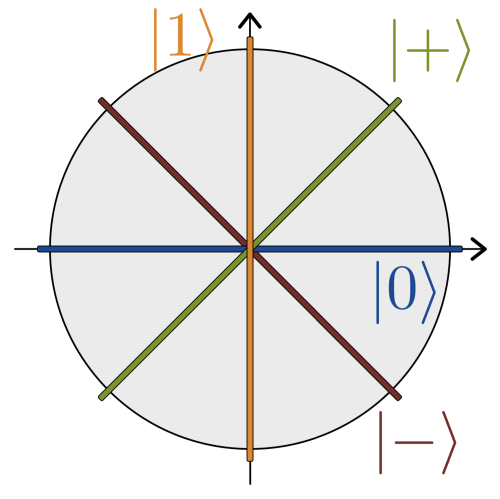Returning to the key features of quantum information, we will deal first with invasive measurements (the principle that quantum information is generally modified when it is measured). To access the value of a qubit, we need to measure it, and this can alter its state. To measure the qubit, we need a measurement basis, which you can visualise as a cross defined by two orthogonal (perpendicular) lines. The two bases we need here are (0, 1) and (+,  –) (see Figure 3). If we measure in a basis, the current state is changed to one of the two orthogonal lines. The probability to be mapped to any of them depends on the angle between the line that describes the qubit and the two orthogonal lines. In the case of our protocol, we only use the angles 0°, 45°, 90°, and 135°, and here the probabilities of measurement are either 0, 1, or ½. In our game, we will use a coin (with 0 being heads and 1 being tails), rather than trigonometry, to measure with respect to our bases.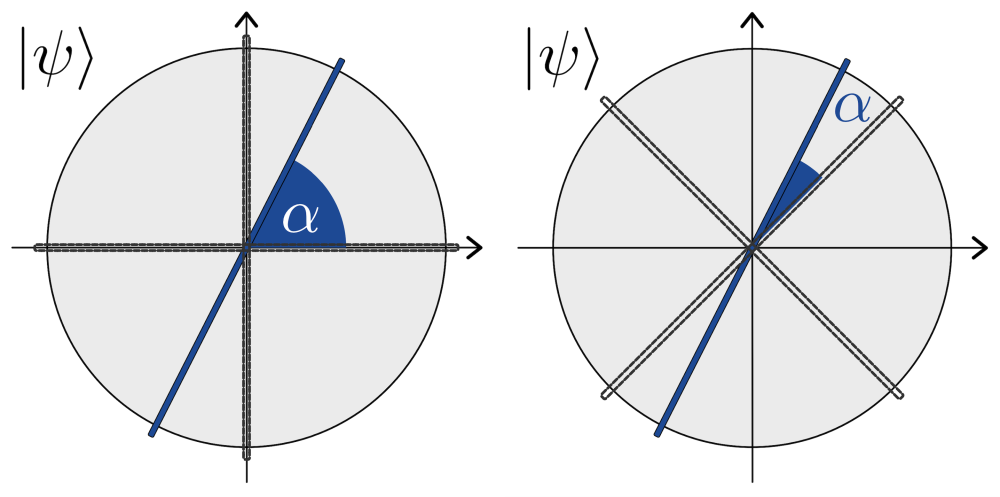The second feature of quantum information is the no-cloning theorem (the principle that quantum information cannot generally be copied). This theorem has a mathematical proof that is outside the scope of this article, but its implication is described by its name. Given an arbitrary quantum state, we cannot simply make a copy of it. This will be important later, as the eavesdropper cannot simply copy the qubits that our two secret-sharers send to each other.

### The BB84 protocol

The BB84 protocol is a quantum key exchange protocol, meaning it allows the exchange of a shared secret bitstring between two people, say Alice and Bob. After exchanging the key, it can be used for secure one-time pad communication or other cryptography protocols. We will give a short description of the necessary steps and describe them as a game played by four students. You will need:

• 8 qubits (a template for a circle with specified angles, a paper arrow, a paper cross, and a brass fastener to mimic the images in the table on the next page — see Figure 4 at the top of the page)

• 4 students (playing the roles of Alice, Bob, Hermes, and Eve)

• 2 coins

Repeat eight times:

1. Alice flips a coin (heads 0, tails 1) to create a random bit. This is the bit intended for the shared key.

2. Alice flips the coin again to randomly decide an encoding basis: (0, 1) or ( + , -).

3. If the random bit is a 0, Alice prepares a qubit in 0 or +. If the random bit is a 1, she prepares a qubit in 1  or – (see Table 1 for possible outcomes).

4. Hermes carries the qubit from Alice to Bob.

5. Bob decides randomly for measurement basis (  0,1) or (  +,-) by flipping a coin.

6. Bob then measures the qubit with the basis from step 5 according to the image. If Alice and Bob’s bases match, Bob measures the same outcome as Alice did. Otherwise, there is a 50 percent chance for each outcome. Hermes helps in these cases by throwing a coin to decide the outcome (see Table 2).

7. Alice and Bob compare which measurement bases they used. If Alice and Bob used the same basis, they include this bit in the key. Otherwise, they discard it.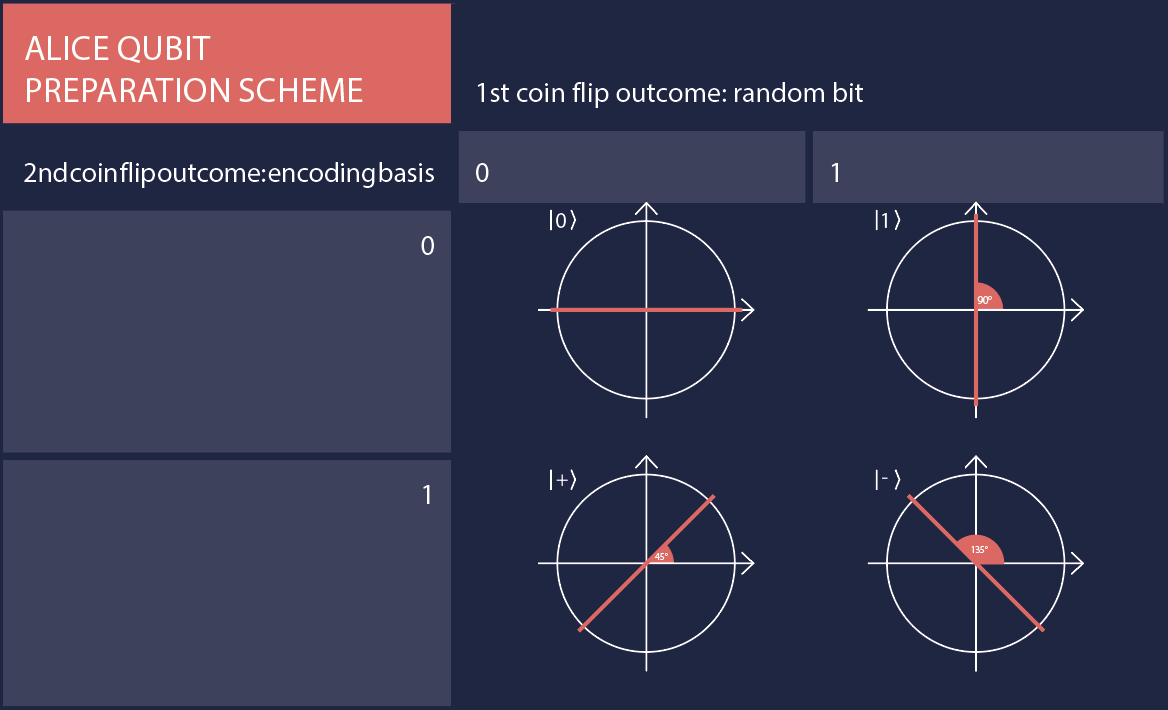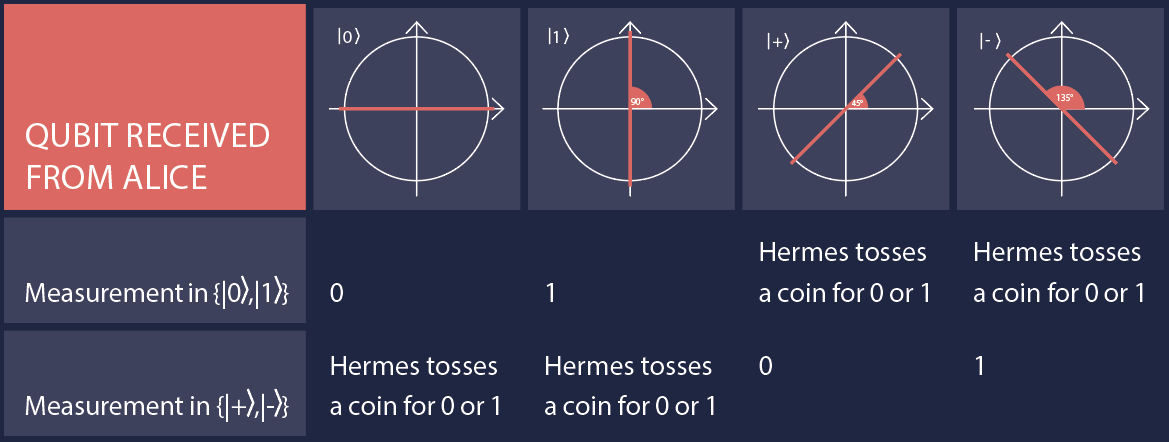The resulting key can now be used for a one-time pad or for any other cryptography protocol.

### Eavesdroppers

Eve, whose role in the protocol has not yet been introduced, is our potential eavesdropper. Her role is to take over the qubits from Hermes at step 4, then do whatever she wants with them before handing them back to Hermes for him to deliver to Bob. Eve can choose to look at each qubit or not. If she does the latter, she cannot gain any information and hence won’t know anything about the qubit’s state. The magic happens as soon as she looks at the qubit, which means that she measures it. To measure the qubit without changing its state, though, she needs to get its basis right (remember: simply copying is not allowed in the quantum information world).

As she knows nothing about the basis Alice used to prepare her qubits, Eve only has a 50 percent chance of guessing correctly. If she does, she can observe the state of the qubit without changing it. But if she chooses the wrong basis, she changes the state of the qubit. After Bob and Alice exchange the bases used for measurements, they can use parts of their remaining qubits to check whether they both measured the same values. If Bob measured on the same basis as Alice, but observed a different outcome, he knows that an eavesdropper has interfered.

In summary, the activity shows a different spin on cryptography, as quantum information allows for different ways of encoding information. By playing this game, students can get a hands-on impression of the protocol and can more easily keep track of the states of the real-life qubits, making these abstract concepts more concrete. They also get a feel for when randomness kicks into quantum information through the coin toss. In our experience, playing BB84 with students can help to deepen their understanding of the core ideas of cryptography.

### Authors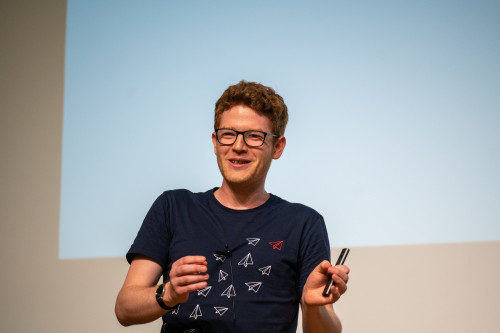Andreas is a PhD student in quantum information science in the Quantum Optics and Statistics group at the University of Freiburg, Germany. He is interested in bringing quantum information to schools.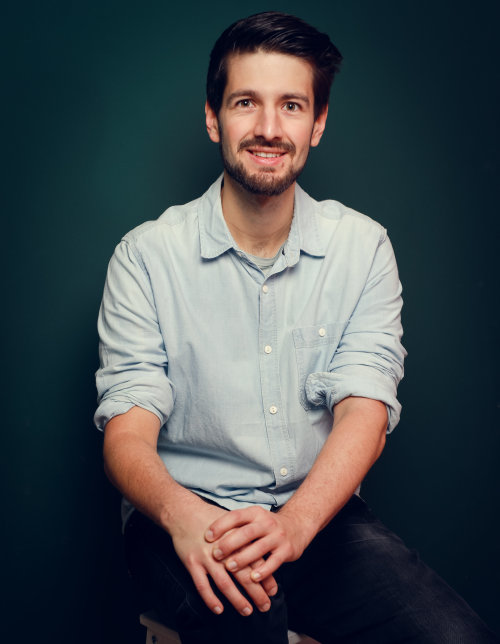Stefan is the quantum education manager at IQM Quantum Computers, exploring ways to make quantum computing accessible to everyone.

## Print

Free - UK only

If you’re a UK-based teacher, volunteer, librarian or something in between, we'll send each issue free to your door.

## Digital

Free

Just want to read the free PDF? Get each new issue delivered straight to your inbox. No fuss and no spam.